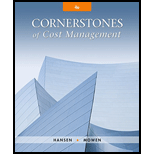# What is the variable cost ratio? The contribution margin ratio? How are the two ratios related?### Cornerstones of Cost Management (C...

4th Edition
Don R. Hansen + 1 other
Publisher: Cengage Learning
ISBN: 9781305970663

#### Solutions

Chapter
Section### Cornerstones of Cost Management (C...

4th Edition
Don R. Hansen + 1 other
Publisher: Cengage Learning
ISBN: 9781305970663
Chapter 16, Problem 6DQ
Textbook Problem
6 views

## What is the variable cost ratio? The contribution margin ratio? How are the two ratios related?

To determine

Describe and relate variable cost ratio and contribution margin ratio.

### Explanation of Solution

Variable Cost: Variable costs refer to the costs that are involved in the production, and vary as per the changes in the volume of production.

Variable Cost Ratio: Variable cost ratio shows the amount of variable cost in the total amount of sales. The formula for variable cost ratio is shown below:

Variable cost ratio = Variable CostsSales

Contribution Margin: The contribution margin refers to the process or the theory that is used to judge the benefit generated from each unit of the goods produced.

Contribution Margin Ratio: The contribution margin ratio shows the amount of difference in the actual sales value and the variable expenses in percentage

### Still sussing out bartleby?

Check out a sample textbook solution.

See a sample solution

#### The Solution to Your Study Problems

Bartleby provides explanations to thousands of textbook problems written by our experts, many with advanced degrees!

Get Started

Find more solutions based on key concepts
Why do economists make assumptions?

Essentials of Economics (MindTap Course List)

Would the yield spread on a corporate bond over a Treasury bond with the same maturity tend to become wider or ...

Fundamentals of Financial Management, Concise Edition (with Thomson ONE - Business School Edition, 1 term (6 months) Printed Access Card) (MindTap Course List)

Why must a signature card be filled out and signed to open a checking account?

College Accounting, Chapters 1-27 (New in Accounting from Heintz and Parry)